Lecture 09: Linearizability and Progress

Last Time

• Sequential consistency:
• consistent with program order
• consistent with sequential specification
• Properties of sequential consistency
• not compositional
• sequentially consistent components do not imply sequential consistency
• nonblocking
• methods can give SC response without waiting for other pending method calls

Today

Linearizable objects and executions

SC makes no reference to invocation/response intervals for method calls

• Why bother with time intervals if we ignore them (execept relative order for single threads)?

Another idea

• Make sure execution is consistent with timing of method calls
• Consider sequential executions consistent with each method call taking effect at some instant during the method call

Same Example, Fewer Options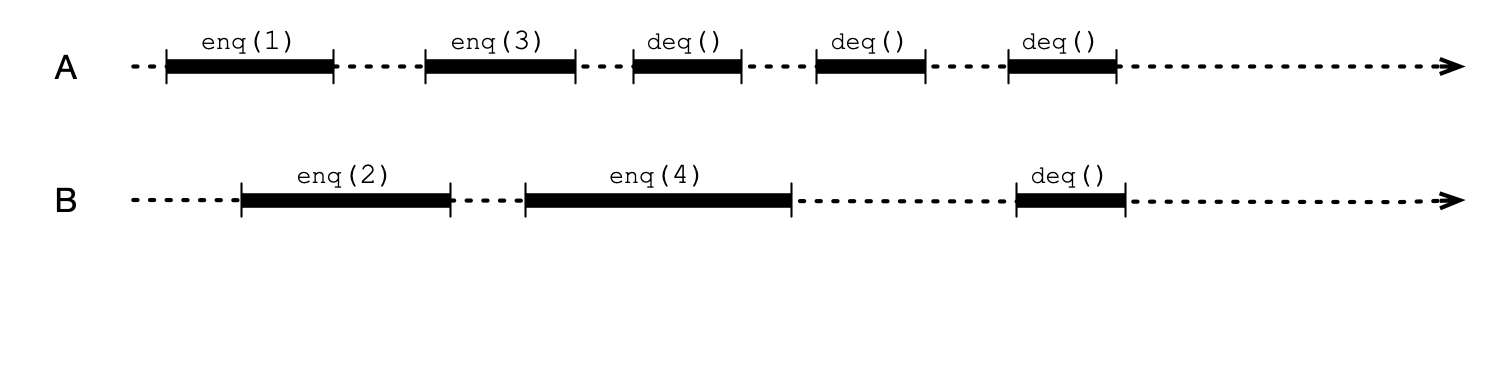Can only change relative order of method calls if they overlap

Linearization Points

A linearization point is a point in a method call where method “takes effect”

• all events after linearization point see effect of method call
• linearization points must be distinct (correspond to some atomic operation)

Example of Linearization Points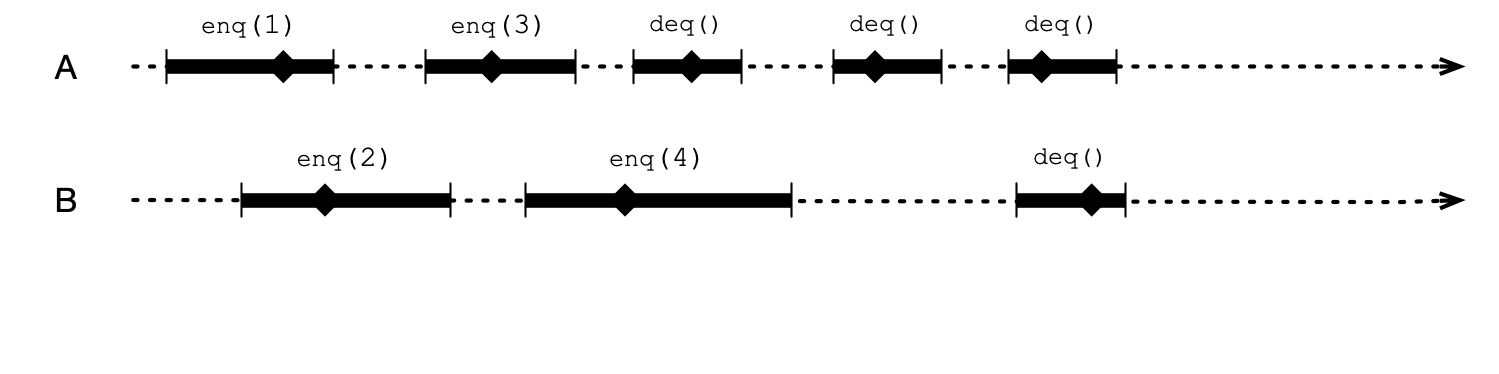Equivalent Sequential Execution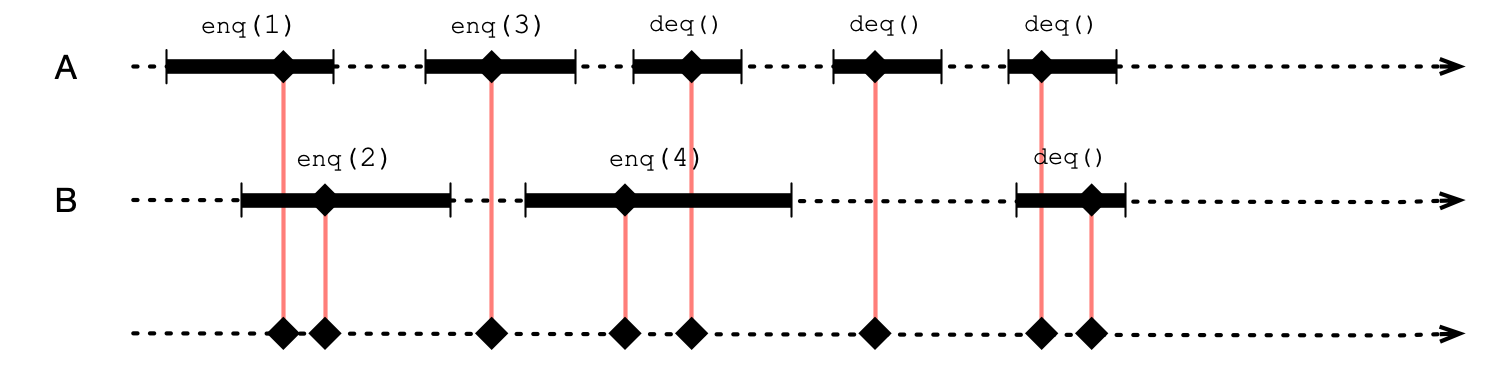An Alternative Sequential Execution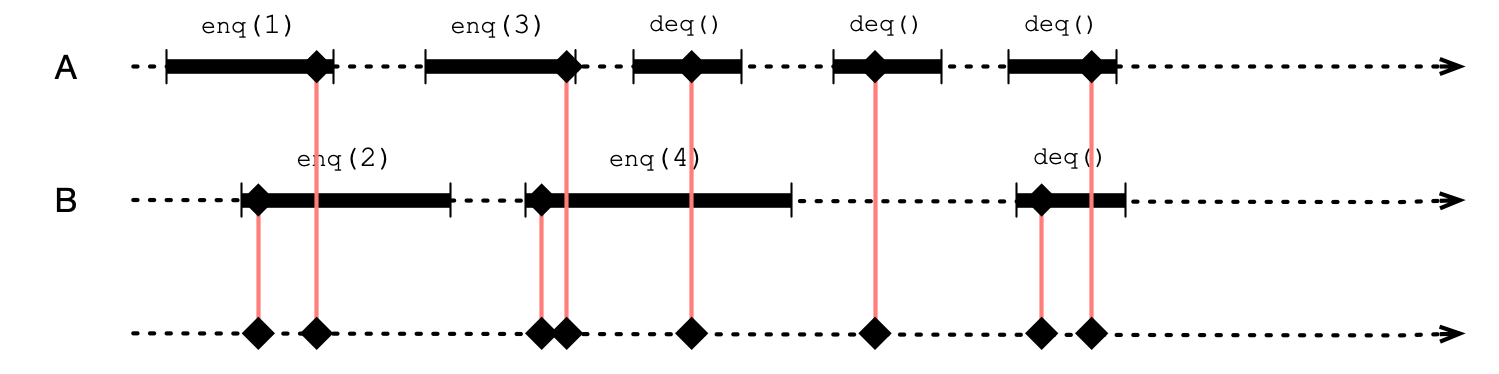Linearizability

A concurrent execution is linearizable if:

• exists a linearization point in each method call such that execution is consistent with sequential execution where method calls occur in order of corresponding linearization points

An implementation of an object is linearizable if:

• it guarantees every execution is linearizable

Comparing Linearizability and SC

1. Does linearizability imply sequential consistency?
2. Does sequential consistency imply linearizability?

Linearizability $\implies$ SC

Must show:

1. Maintains program order
2. Satisfies sequential specification

SC $\nRightarrow$ Linearizability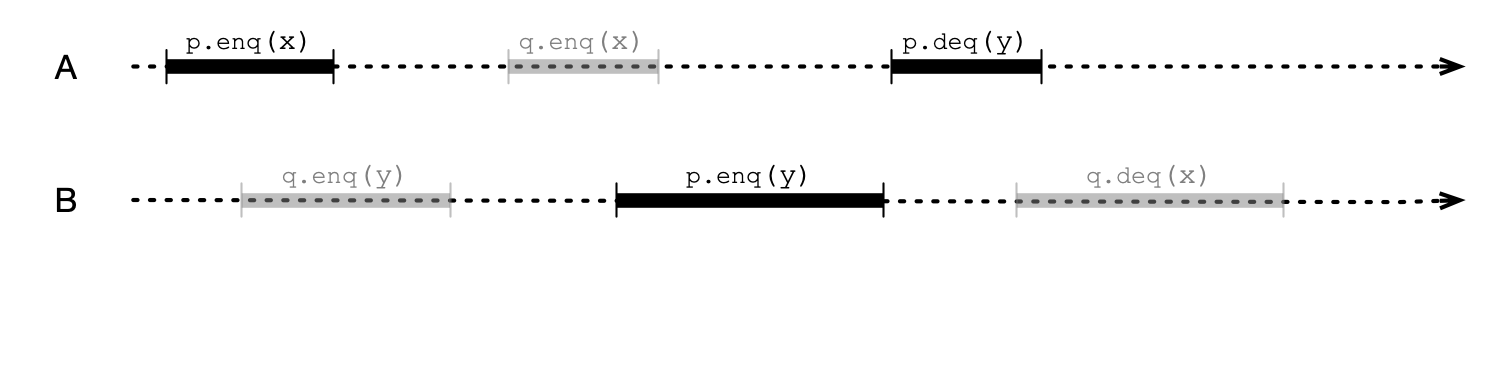Compositionality

Recall this execution with 2 queues (not sequentially consistent)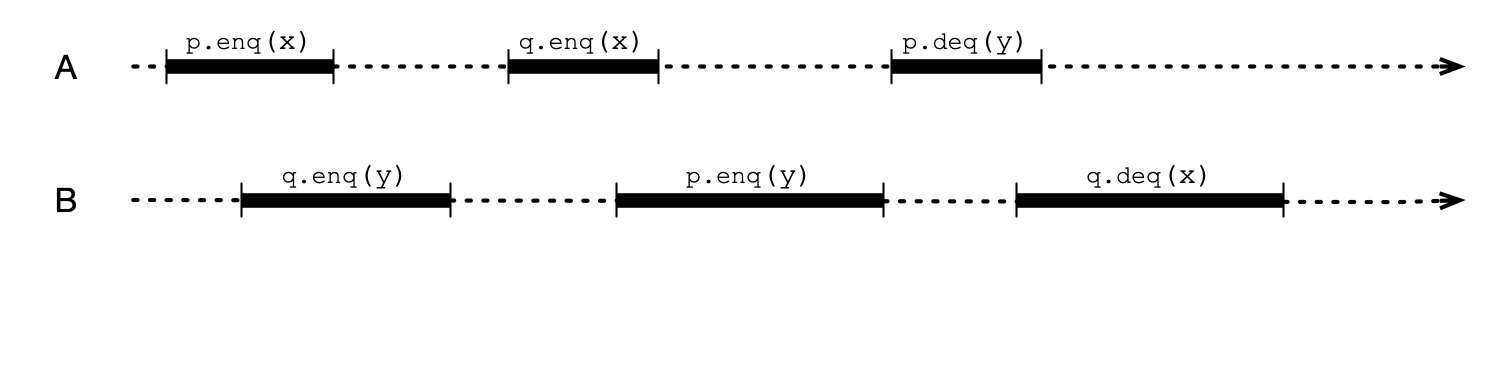But…

p and q are not linearizable in this execution!Two More Questions

1. Are linearizable objects compositional?
2. Is linearizability a nonblocking condition?

Coming Up

Implementations!

• locks
• data structures

Homework Questions

Question 1

Suppose $$n$$ threads call the visit() method of a Bouncer object. Argue that the following hold:

1. At most one thread gets the value STOP.
2. At most $$n - 1$$ threads get the value DOWN.
3. At most $$n - 1$$ threads get the value RIGHT.

Question 2

Consider a 2D triangular array of Bouncer objects arranged as follows: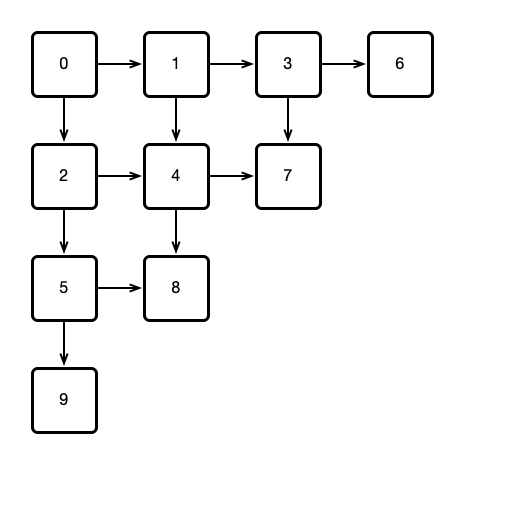Question 2, Continued

Suppose:

• All threads start by calling visit() on Bouncer 0.
• Whenever a thread visits a Bouncer, if the Bouncer returns STOP, the thread adopts the number of the Bouncer as its ID.
• If DOWN is returned, the thread then visits the Bouncer below; if RIGHT is returned, the thread visits the Bouncer to the right.
1. Show that for a sufficiently large array of Bouncers, every thread will eventually STOP at some Bouncer, thereby adopting its ID.
2. If the number $$n$$ of threads is known in advance, how many Bouncers are required to ensure that all threads adopt an ID?